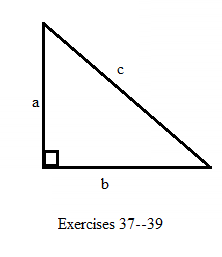# In Exercises 37-39, c 2 = a 2 + b 2 . In the right triangle, find b if a = 6 a n d c = 10 .### Elementary Geometry for College St...

6th Edition
Daniel C. Alexander + 1 other
Publisher: Cengage Learning
ISBN: 9781285195698

#### Solutions

Chapter
Section### Elementary Geometry for College St...

6th Edition
Daniel C. Alexander + 1 other
Publisher: Cengage Learning
ISBN: 9781285195698
Chapter A.5, Problem 38E
Textbook Problem
1 views

## In Exercises 37-39, c 2 = a 2 + b 2 .In the right triangle, find b if a = 6     a n d     c = 10 .To determine

To find:

To find b if a=6andc=10.

### Explanation of Solution

Consider the following equation,

c2=a2+b2.

The diagrammetic representation is given below,

Substitute the values of a=6andc=10 in the above equation to get the following,

c2=a2+b2102=62+b2100=36+

### Still sussing out bartleby?

Check out a sample textbook solution.

See a sample solution

#### The Solution to Your Study Problems

Bartleby provides explanations to thousands of textbook problems written by our experts, many with advanced degrees!

Get Started

Find more solutions based on key concepts
Differentiate the function. f(x) = 5.2x + 2.3

Calculus: Early Transcendentals

a. Let f, g, and h be functions. How would you define the "sum of f, g, and h? b. Give a real-life example invo...

Applied Calculus for the Managerial, Life, and Social Sciences: A Brief Approach

In Problem 1-10, find the real solutions to each quadratic equation.

Mathematical Applications for the Management, Life, and Social Sciences

Explain why the formula for sample variance is different from the formula for population variance.

Essentials of Statistics for The Behavioral Sciences (MindTap Course List)

True or False: (x + y)y″ + (x − 10)y′ + xy + 10 = 0 is homogeneous.

Study Guide for Stewart's Multivariable Calculus, 8th

Rewrite 10x2xdx using a trigonometric substitution. a) cos10sind b) 110sincos2d c) 10cos2sind d) 10sincosd

Study Guide for Stewart's Single Variable Calculus: Early Transcendentals, 8th